Published

# FDM Printer Module Control

UNCC_ME_PRINT (UNCC Senior Design Project). Actuating Creality Extruder Module for FDM Printing

IntermediateWork in progress2 hours21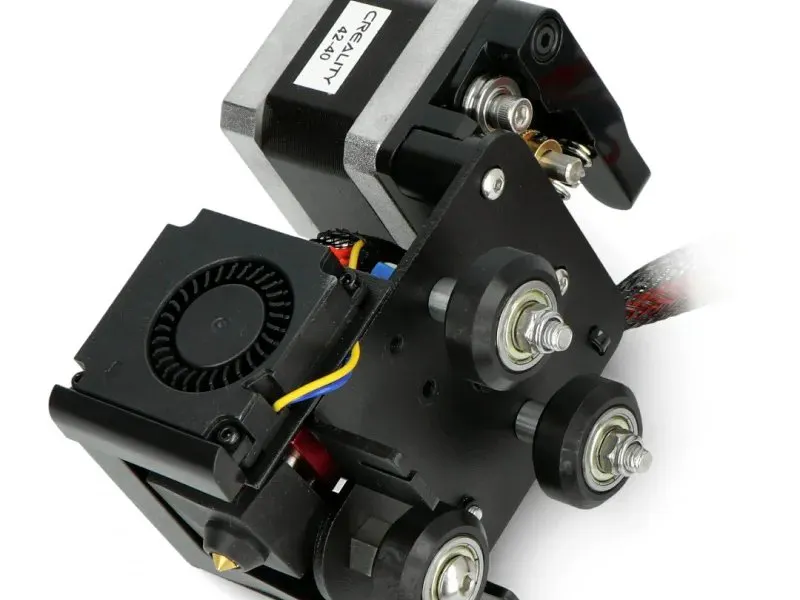## Things used in this project

### Hardware components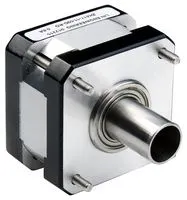Stepper Motor, Bipolar
×1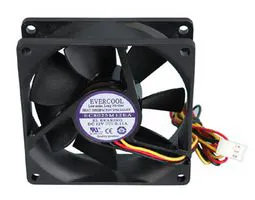Axial Fan, 12 V
×1Arduino UNO
×1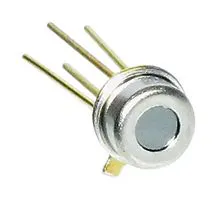Thermopile IR Sensor, TS318-1B0814
×1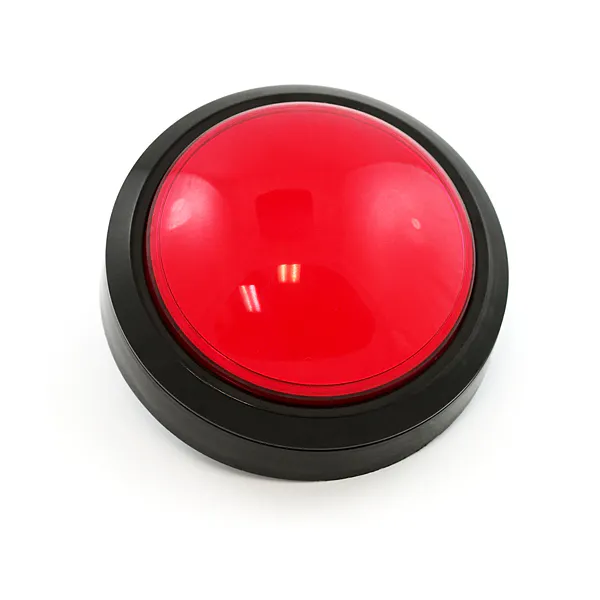SparkFun Big Red Dome Button
×1

## Schematics

### seniordesignschematic_sRV5qoZSbA.fzz

Extruder Control Schematic

### Wiring Diagram

Electric Configuration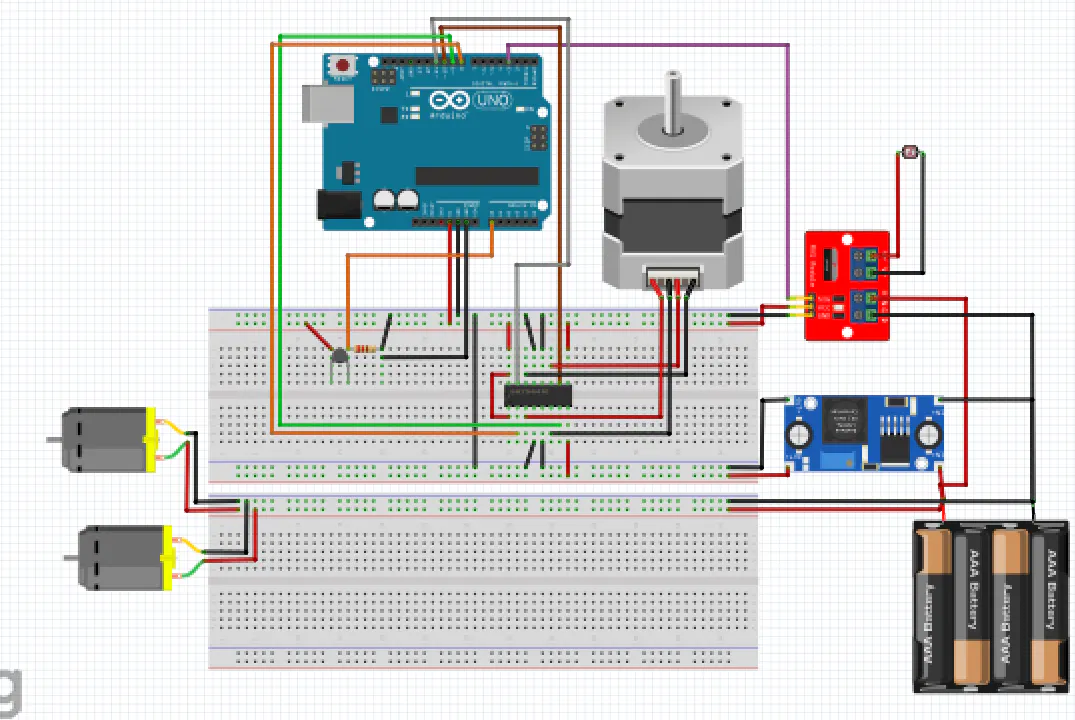## Code

### Creality Extruder Control Program

Arduino
Actuates Hot-End and Stepper motor. Gets data from thermistor and button.
```/*
Marcus Neacsu
UNCC_ME_PRINT - Creality Extruder Control
May 11, 2023
Credits
- Thermistor: https://projecthub.arduino.cc/Marcazzan_M/81ae74bf-ba05-4813-ba39       -572254cf3942
- Stepper: https://docs.arduino.cc/learn/electronics/stepper-motors
*/
#include <Stepper.h>

int stepsPerRevolution 200;

/*thermistor paramaters*/
#define THERMISTOR_PIN A0
//Resistance value
const long Resistor = 100000.0;
//Room temperature resistance value of thermistor
const long TempResistance = 100000.0;
//thermal coefficient
const int ThermoCoeff = 3950;

//pins for the stepper motor on the Arduino
Stepper stepper(stepsPerRevolution , 8, 9, 10, 11);

//melting temperature for PLA
float maxtemp = 200.0;

/*CHANGE THESE VALUES TO TUNE PID CONTROLLER*/
float kp = 12;
float ki = 0;
float kd = 0;

/*dont change these*/
float Perror = 0;
float Ierror = 0;
float Pasterror = 0;
float Derror = 0;

void setup() {
// Begin the Serial monitor to track the temperature
Serial.begin(9600);
//set thermistor pin as a input
pinMode(THERMISTOR_PIN,INPUT);
//set the button as input
pinMode(4, INPUT_PULLUP);
//set hotend as output
pinMode(5, OUTPUT);
//set stepper speed
stepper.setSpeed(20);
}

void loop() {
//read the analog value for temperature
//convert analog to voltage amount (millivolt for more percision (map only does int math))
float tempvoltageMV = map(analogtemp, 0.0, 1023.0, 0.0, 5000.0);
//convert to volts
float tempvoltage = tempvoltageMV / 1000.0;
float VoltageOut = (5.0 - tempvoltage);
//convert volts to resistance
float thermistorResistance = tempvoltage / (VoltageOut/(Resistor));
//convert the resistance value to temperature
float ln = log(thermistorResistance / TempResistance);
float thermistortemperatureK = (1.0 / ((ln / ThermoCoeff) + (1.0 / (25.0+273.15))));
//convert K to C
float thermistortemperatureC = thermistortemperatureK - 273.15;
//convert C to F
float thermistortemperatureF = (thermistortemperatureC *1.8) +32.0 ;
//print the temperature
Serial.println(thermistortemperatureF);

//Potential Controller for hotend
Perror = abs(maxtemp - thermistortemperatureF);
float heaterpower = (kp * Perror);
//cap at the max temperature
if (thermistortemperatureF <= maxtemp) {
analogWrite(5, heaterpower);
}
else{
analogWrite(5,0);
}
//if button is pressed down
if (button == 0){
//acutate the stepper motor
stepper.step(-10);
}
delay(100);
}
```

## Credits

### Marcus Neacsu

1 project • 2 followers